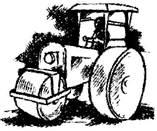# A road roller takes 750 complete revolutions to move once over to level a road. Find the area of the road if the diameter of a road roller is 84 cm and length 1 m.Given

Diameter of road roller, d = 84 cm

Radius of road roller, r = d/2 = 84/2 = 42 cm

Length of road roller, h = 1 m = 100 cm

Find out

We have to find out the area of the road

Solution

We know that the formula for Curved surface area of road roller = 2πrh

= 2×(22/7)×42×100 = 26400

The curved surface area of road roller is 26400 cm2

Area covered by road roller in 750 revolutions

= 26400×750cm2

= 1,98,00,000cm2

= 1980 m2 [∵ 1 m2= 10,000 cm2]

Hence the area of the road is 1980 m2.